Next: Aligning Children Up: Generating the Edit Previous: Generating the Edit

## Outline of Algorithm

The algorithm is most easily explained as consisting of the five phases that we describe below. We use our running example from Figure 1. We are required to find a minimum cost edit script that transforms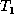into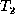, given the matching M shown by the dashed lines in the figure.

The Update Phase: In the update phase, we look for pairs of nodes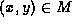such that the values at nodes x and y differ. For each such pair (in any order) we add the edit operation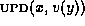to E (recall that for a node x,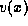denotes the value of x), and we apply the update operation to. At the end of the update phase, we have transformedsuch that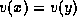for every pair of nodes.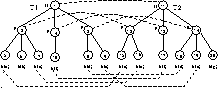Figure 4: Running example: after align phase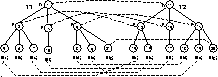Figure 5: Running example: after insert phase

The Align Phase: Let the partner of a node denote the node to which it is matched (by a given matching). Suppose. We say that the children of x and y are misaligned if x has matched children u and v such that u is to the left of v inbut the partner of u is to the right of the partner of v in. In Figure 1, the children of the root nodes 1 and 11 are misaligned. In the align phase we check each pair of matched internal nodes(in any order) to see if their children are misaligned. If we find that the children are misaligned, we append move operations to E to align the children. We explain how the move operations are determined in Section 4.2 below. In our running example, we append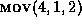to E, and we apply the move operation to. The newis shown in Figure 4.

The Insert Phase: We assume, without loss of generality, that the roots ofandare matched in M.In the insert phase, we look for an unmatched node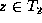such that its parent is matched. Suppose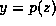(i.e., y is the parent of z) and y's partner inis x. We create a new identifier w and append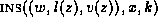to E. The position k is determined with respect to the children of x and z that have already been aligned with respect to each other; details are in Section 4.3. We also apply the insert operation toand add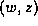to M. In our running example we append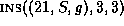. The transformedand the augmented M are shown in Figure 5. At the end of the insert phase, every node inis matched but there may still be nodes inthat are unmatched.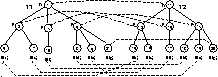Figure 6: Running example: after delete phase

The Move Phase: In the move phase we look for pairs of nodessuch that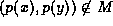. (Recall from Section 3.1 that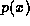denotes the parent of x.) Suppose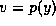. We know that at the end of the insert phase, v has some partner u in. We append the operation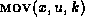to E, and we apply the move operation to. Here the position k is determined with respect to the children of u and v that have already been aligned, as in the insert phase. At the end of the move phaseis isomorphic toexcept for unmatched nodes in. In our running example, we do not need to perform any actions in this phase.

The Delete Phase: In the delete phase we look for unmatched leaf nodes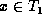. For each such node we append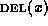to E and apply the delete operation to. (Note that this process will result in a bottom-up delete---descendents will be deleted before their ancestors.) At the end of the delete phaseis isomorphic to, E is the final edit script, and M is the total matching to which E conforms. Figure 6 shows the trees and the matching after the delete phase.Next: Aligning Children Up: Generating the Edit Previous: Generating the Edit

Sudarshan S. Chawathe
Wed Jun 19 08:22:34 PDT 1996